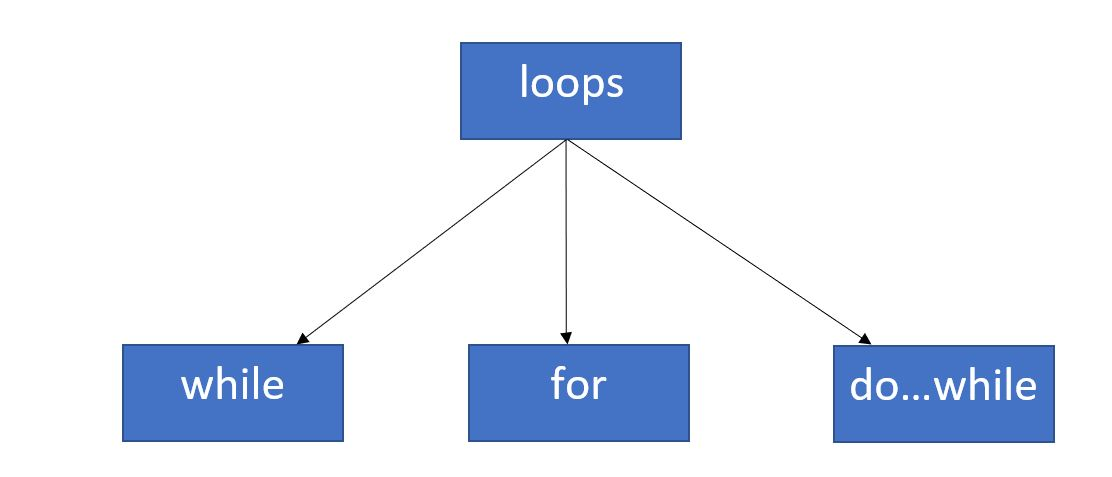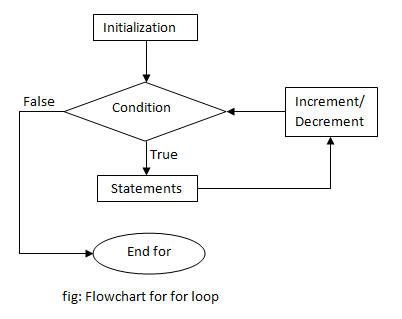×

Search anything:

# for loop in C++

#### C++ for loop Software EngineeringGet this book -> Problems on Array: For Interviews and Competitive Programming

Reading time: 20 minutes | Coding time: 2 minutes

Loop is a programming concept which is natively supported by nearly all programming languages. Loops are used to repeat a particular coding task where each repetition follows a particular pattern which can be incorporated in a code. Example of such a task is printing integers from 1 to 10. The repetition is that we are printing integers and the pattern is that we start from 1 and increment by 1 each step. In this article, we will take a look at loops in C++.

The concept of loops comes into picture when we need to implement/print a particular block/statement more than one time till a particluar condition is specified. We have different types of loops :

Entry Controlled loop : Where the entry of the loop is controlled that is we have a condition at the starting of the loop. Example : while loop

Exit Controlled loop : In this type of loop the exit from the loop is controlled that is we have a condition at the end of the loop. Example : do..while loopIn this particular section we are going to discuss for loop.

Syntax :

``````for (initializationStatement; testExpression; updateStatement)
{
// codes
}
``````

Where :

initializationStatement is the statement where we to initialize the loop counter to some value.
Example : int i=0;

testExpression in this expression we have to test the condition. If the condition is to true then we will execute the body of loop otherwise we will exit from the for loop.
Example : i<=10;

updateStatement after executing loop body this statement is encountered and is incremented/decremented depending on the statement.
Example : i++

### Order of the excution of for loop

1)First we intialize the loop counter, In C++ it can be declared as well as intialized in the intializationStatement.

2)Then the condition is checked i.e testExpression is evaluated.

3)After this we enter the loop and print/execute the statement(s)/block.

4)Then the value of the loop counter in incremented/decremented depending on the updateStatement.

5)Now we will repeat Step 2-4 till the testExpression condition is violated.### Example

In this example, we will write a program to print number from 1 to 10.

``````#include<iostream>
using namespace std;
int main()
{
int i;

for(i=0;i<10;i++)
{
cout<<i+1<<"\n";
}
return 1;
}
``````

### Difference between for and while loop

1. Declaration:
``````  for(initializationStatement; testExpression; updateStatement)
{
// code
}
While(testExpression)
{
//code
}
``````
1. Condition:
If condition is not mentioned then the loop will iterate infinite times in for loop. If condition is not mentioned then it provides compilation error in while loop.
2. Iteration Statement:
The iteration statement is written on the top hence executes only after all statements in for loop are executed.The statement can be mentioned anywhere in the while loop.
3. Use:
for loop isused when we already know the number of iterations but while loop is used when number of iterations are not exactly known.

### Questions

See this C++ code:

``````#include<iostream>
using namespace std;
int main()
{
int i=0,x=0;

for(i=1;i<10;i*=2)
{
x++;
cout<<x;
}
cout<<x;
return 0;
}
``````

#### What will be the output of the above code?

12344
1234567899
12345678910
123455
In postfix(i++) the value is first stored and then incremented.#### Harshita Sahai

Maintainer at OpenGenus | Previously Software Developer, Intern at OpenGenus (June to August 2019) | B.Tech in Information Technology from Guru Gobind Singh Indraprastha University (2017 to 2021)